Students must start practicing the questions from CBSE Sample Papers for Class 10 Maths with Solutions Set 11 are designed as per the revised syllabus.

## CBSE Sample Papers for Class 10 Maths Standard Set 11 with Solutions

Time Allowed: 3 Hours
Maximum Marks: 80

General Instructions:

1. This Question Paper has 5 Sections A-E.
2. Section A has 20 MCQs carrying 1 mark each
3. Section B has 5 questions carrying 02 marks each.
4. Section C has 6 questions carrying 03 marks each.
5. Section D has 4 questions carrying 05 marks each.
6. Section E has 3 case based integrated units of assessment (04 marks each) with sub-parts of the values of 1, 1 and 2 marks each respectively.
7. All Questions are compulsory. However, an internal choice in 2 Qs of 5 marks, 2 Qs of 3 marks and 2 Questions of 2 marks has been provided. An internal choice has been provided in the 2 marks questions of Section E
8. Draw neat figures wherever required. Take π =22/7 wherever required if not stated.

Section – A
(Section A consists of 20 questions of 1 mark each.)

Question 1.
If di = xi – a, $$\sum_{i=1}^n f_i=25, a=250, \bar{x}=250$$ then $$\sum_{i=1}^n f_i d_i$$ is:
(a) 0
(B) – 2
(c) 20
(d) 50
(a) 0

Explanation:
It is given that:
di = xi – a, $$\sum_{i=1}^n f_i=25, a=250, \bar{x}=250$$ then $$\sum_{i=1}^n f_i d_i$$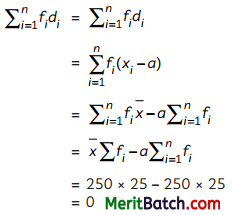Question 2.
The zeroes of the quadratic polynomial 3x2 + 10x + 8 are:
(a) -2 and $$\frac{4}{3}$$
(b) 2 and –$$\frac{4}{3}$$
(c) – 2 and –$$\frac{4}{3}$$
(d) 2 and $$\frac{4}{3}$$
(c) – 2 and –$$\frac{4}{3}$$

Explanation:
p(x) = 3x2 + 10x + 8
= 3x2 + 6x + 4x + 8
= 3x(x + 2) + 4(x + 2)
= (3x + 4) (x + 2)
For finding zeroes, put p(x) = 0
(3x + 4) (x + 2) = 0
x = – $$\frac{4}{3}$$, -2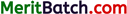Question 3.
If in two triangles ∆ABC and ∆PQR, ∠A = ∠Q and ∠R = ∠B, then which of the following is not true?
(a) $$\frac{B C}{P R}=\frac{A C}{P Q}$$
(b) $$\frac{A B}{P Q}=\frac{B C}{R P}$$
(c) $$\frac{A B}{Q R}=\frac{A C}{P Q}$$
(d) $$\frac{B C}{R P}=\frac{A B}{Q R}$$
(b) $$\frac{A B}{P Q}=\frac{B C}{R P}$$
Explanation: Given : ∠A = ∠Q,
∠B = ∠R∴ ∆ABC ~ ∆QRP
∴ $$\frac{A B}{P Q}=\frac{B C}{R P}=\frac{C A}{P Q}$$

Question 4.
The angle of elevation of the top of a tower at a point on the ground 40 m away from the foot of the tower is 45°. Then the height of the tower (in metres) is:
(a) $$\frac{50}{\sqrt{2}}$$ m
(b) $$\frac{50}{\sqrt{3}}$$ m
(c) 50$$\sqrt{3}$$ m
(d) 40 m
(d) 40 m
Explanation:
Here, AB is a tower and C is a point on the ground 40 m from the foot of the tower.In ∆ABC
tan 45° = $$\frac{A B}{B C}$$
1 = $$\frac{A B}{40}$$
AB =40 m

Question 5.
The value of ‘c’ for which the pair of linear equations cx – y = 2 and 6x – 2y = 3 will have infinitely many solutions, is:
(a) 3
(b) -3
(c) -12
(d) no value
(d) no value

Explanation:
Given, pair of linear equations are
cx – y = 2 and 6x – 2y = 3
Here, a1 = c, b11 = -1, c1 = -2
a2 = 6, b2 = -2, c2 = -3
∴ $$\frac{a_1}{a_2}=\frac{b_1}{b_2}=\frac{c_1}{c_2}$$
$$\frac{a_1}{a_2}=\frac{c}{6}, \frac{b_1}{b_2}=\frac{-1}{-2}=\frac{1}{2}, \frac{c_1}{c_2}=\frac{-2}{-3}=\frac{2}{3}$$
Here,
$$\frac{b_1}{b_2} \neq \frac{c_1}{c_2}$$
∴ c does not has any value.

Question 6.
While computing mean of a grouped frequency distribution, we assume that the frequencies are:
(a) evenly distributed over all the classes
(b) centered at the class marks of the classes
(c) centered at the lower limits of the classes
(d) scentered at the upper limits of the classes
(b) centered at the class marks of the classes

Explanation:
While computing mean of grouped data, we assume that the frequencies are centred at the class marks of the class.Question 7.
The line segment joining the point (7, -6) and (3, 4) is divided by a point in the ratio 1 : 2 internally. The quadrant in which this point is:

Explanation:
Using the section formula, if a point (x, y) divides the line joining the points
(x1, y1) and (x2, y2) internally in the ratio m : n, then (x, y)
= $$\frac{m x_2+n x_1}{m+n}, \frac{m y_2+n y_1}{m+n}$$
put (x1, yi) = (7, -6) and (x2, y2) = (3, 4) and m = 1, n = 2 in section formula, we get the point
$$\left(\frac{1(3)+2(7)}{1+2}, \frac{1(4)+2(-6)}{1+2}\right)=\left(\frac{17}{3},-\frac{8}{3}\right)$$
Hence, point lies in IV quadrant.

Question 8.
If a and b are the zeroes of the polynomial 5x2 – 7x + 2, then the sum of their reciprocals is:
(a) $$\frac{7}{2}$$
(b) $$\frac{7}{5}$$
(c) $$\frac{2}{5}$$
(d) $$\frac{14}{25}$$
(a) $$\frac{7}{2}$$

Explanation:
Since, a and b are the zeroes of the polynomial 5x2 – 7x + 2.
α + ß = $$\frac{7}{2}$$, αß = $$\frac{2}{5}$$
Now $$\frac{1}{α}$$ + $$\frac{1}{ß}$$ = $$\frac{α+ß}{αß}$$
= $$\frac{7/5}{2/5}$$ = $$\frac{7}{2}$$

Question 9.
From a point A which is at a distance of 13 cm from the centre O of a circle of radius 5 cm, the pair of tangents AB and AC to the circle are drawn. Then, the area of the quadrilateral ABOC is:
(a) 30 sq cm
(b) 32.5 sq cm
(c) 60 sq cm
(d) 65 sq cm
(c) 60 sq. cm

Explanation:Area of quadrilateral ABOC = ar(∆ABO) + ar(∆ACO)
= $$\frac{1}{2}$$ × AB × OB + $$\frac{1}{2}$$ × AC × OC
(∵ Tangent is ⊥r to radius at the point of contact)

In ∆AOB, by pythagoras theorem
AB = $$\sqrt{OA^2 + OB^2 }$$
= $$\sqrt{13^2 + 5^2 }$$
= $$\sqrt{169-25 }$$
= 12 cm
Now, AB = AC (length of tangents are equal from external)
= $$\frac{1}{2}$$ × 5 × 12 + $$\frac{1}{2}$$ × 5 × 12
= 60 cm2Question 10.
If the LCM of p and 18 is 36 and the HCF of p and 18 is 2, then the value of p is:
(a) 1
(b) 2
(c) 3
(d) 4
(d) 4

Explanation:
LCM of p and 18 = 36
HCF of p and 18 = 2
∴ Product of numbers = LCM × HCF of two numbers
⇒ p × 18 = 2 × 36
⇒ p = $$\frac{2 × 36}{18}$$ = 4

Question 11.
If (a/3, 4) is the mid-point of the segment joining the points P(-6, 5) and R(-2, 3), then the value of‘a’ is:
(a) 12
(b) -6
(c) -12
(d) -4
(c) -12

Explanation:
Reason:
midpoint = $$\frac{-6-2}{2},\frac{5+3}{2}$$
($$\frac{a}{3}$$, 4) = (-4, 4)
∴ $$\frac{a}{3}$$ = -4
⇒ a = -12

Question 12.
If 1 is a common root of ax2 + ax + 2 = 0 and x2 + x + b = 0, then a: bis:
(a) 1: 2
(b) 2 :1
(c) 1: 4
(d) 4 :1
(a) 1: 2

Explanation:
Given, equations are ax2 + ax + 2 = 0 and x2 + x + b = 0
1 is a common root of both the equations.
Therefore, it will satisfy both the equations
P(1) = a(1)2 + a x 1 + 2 = 0
⇒ 2a + 2 = 0
⇒ a = -1
and P'(1)
⇒ (1)2 + 1 + b = 0
⇒ 2 + b = 0
b = -2
So, a : b = -1 : – 2 = 1 : 2

Question 13.
In two triangles AABC and APQR, ∠B = ∠Q, ∠R = ∠C and AB = 3PQ, what can you say about the two triangles?
(a) congruent but not similar
(b) similar but not congruent
(c) neither congruent nor similar
(d) congruent as well as similar
(b) similar but not congruent

Explanation:
Given:∠B = ∠Q
∠C = ∠R
AB = 3 PQ
Then, ABC ~ PQR (by AA congrency criterion)

Question 14.
If the circumference of a circle is equal to that of a square, then the ratio of their areas is:
(a) 22:7
(b) 14:11
(c) 7: 22
(d) 11:14
(b) 14 : 11

Explanation:
Given:
Circumference of circle = Perimeter of square
2πr = 4a
when, ‘r’ is the radius of the circle and ‘d’ is the side of the square.
∴ 2 × $$\frac{22}{7}$$ × r = 4a
⇒ a = $$\frac{11}{7}$$r …….(i)
$$\frac{Area of circle }{Area of square}$$ = $$\frac{πr^2}{a}$$
= $$\frac{22×r^2}{7(\frac{11}{7})^2r^2}$$
= $$\frac{22}{7}$$ ×$$\frac{49}{121}$$
= $$\frac{14}{11}$$Question 15.
A tangent, marked XAY, is drawn to a circle with a diameter of AB and a radius on 5 cm at one end. The length of the chord CD parallel to XY and at a distance 8 cm from A, is:
(a) 8 cm
(b) 6 cm
(c) 5 cm
(d) 4 cm
(a) 8 cm

Explanation:
XAY || CED
∠XAO = 90° (since, tangent is ⊥r to the radius)Now, ∠OED = 90° (As AY || CED)
∴ CE = ED (As ⊥ from centre bisects the chord)
∴ In ∆OED,
OD2 = OE2 + ED2
52 = 32 + ED2
⇒ ED2 = 25 – 9
= 16
ED = 4 cm
Then, CD = 2 ED = 8 cm

Question 16.
The area of a circle that can be inscribed in a square of side 6 cm is:
(a) 36π sq. cm
(b) 18π sq. cm
(c) 12π sq. cm
(d) 9π sq. cm
(d) 9π sq. cm

Explanation:
Here, PQRS is a square of side 6 cm and a circle is incribed in it.Diameter of circle = 6 cm
Radius of circle (r) = 3 cm
Area of circle = πr2
= p(3)2
= 9π sq. cm

Question 17.
The king, queen, and jack of clubs are removed from a 52-card deck before it is properly shuffled. The probability that a king will be dealt if a card is picked at random from the remaining cards is:
(a) $$\frac{5}{48}$$
(b) $$\frac{3}{52}$$
(c) $$\frac{4}{49}$$
(d) $$\frac{3}{49}$$
(d) $$\frac{3}{49}$$

Explanation:
Here, King, queen and jack of club are removed from the Deck of 52 playing cards.
So remaining cards in deck = 52 – 3 = 49
Total number of outcomes = 49
We know that there are 4 Kings in a deck, After removing a king of club we left with 3 kings.
Let E2 = event of getting a king
Number of favourable outcomes to E2 = 3
Required probability P(E2) = $$\frac{3}{49}$$Question 18.
The next term of the AP : $$\sqrt{8}$$, $$\sqrt{18}$$, $$\sqrt{32}$$, …….. is:
(a) $$\sqrt{65}$$
(b) $$\sqrt{60}$$
(c) $$\sqrt{50}$$
(d) $$\sqrt{55}$$
(c) $$\sqrt{50}$$

Explanation: Given, AP is $$\sqrt{8}$$, $$\sqrt{18}$$, $$\sqrt{32}$$, ……..
or 2$$\sqrt{2}$$, 3$$\sqrt{2}$$, 4$$\sqrt{2}$$ ……………
Then, fist term a = 2$$\sqrt{2}$$
common diffrence d = 3$$\sqrt{2}$$ – 2$$\sqrt{2}$$
= $$\sqrt{2}$$
Then, next term = 4$$\sqrt{2}$$ + $$\sqrt{2}$$
= 5$$\sqrt{2}$$
= $$\sqrt{50}$$

DIRECTION: In the question number 19 and 20, a statement of assertion (A) is followed by a statement of reason (R).
Choose the correct option as:
(a) Both assertion (A) and reason (R) are true and reason (R) is the correct explanation of assertion (A)
(b) Both assertion (A) and reason (R) are true and reason (R) is not the correct explanation of assertion (A)
(c) Assertion (A) is true but reason (R) is false.
(d) Assertion (A) is false but reason (R) is true.

Question 19.
Statement A (Assertion): The angle of elevation of the sun is 45 degrees if the length of a vertical pole’s shadow equals its height. Statement R (Reason): According to pythagoras theorem, h2= l2 + b2 , where h = 1 hypotenuse, l = length and b = base.
(b) Both assertion (A) and reason (R) are true, but reason (R) is not the correct explanation of assertion (A).

Explanation: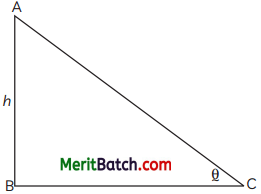In right angle ∆ABC, AB represents the vertical pole and BC represents the shadow on the ground. θ represents angle of elevation the sun.
tan θ = $$\frac{h}{x}$$h
But length of shadow = height of the tower, i.e. h = x
tan θ = $$\frac{h}{h}$$ = 1
tan θ = tan 45º
θ = tan 45º
Thus, angle of elevation of the sun = 45º
and pythagoras theorem, h2 = l2 + b2Question 20.
Statement A (Assertion): The point (-1, 6) divides the line segment joining the points (-3,10) and (6, – 8) in the ratio 2 : 7 internally.
Statement R (Reason): If m : n is the ratio in which point p divides a line segment A(x1, y1) and (x2, y2). Then coordinates of p are p(x,y) = $$\left(\frac{m_1 x_2+m_2 x_1}{m_1+m_2}, \frac{x_1 y_2+x_2 y_1}{x_1+x_2}\right)$$.
(a) Both assertion (A) and reason (R) are true, but reason (R) is not the correct explanation of assertion (A).

Explanation:
By section formula, (x, y) = $$\left(\frac{m x_2+n x_1}{m+n}, \frac{m y_2+n y_1}{m+n}\right)$$
m = k, n = 1
Thearefore, -1 = $$\frac{6K-3}{K+1}$$
-K – 1 = 6K – 3
7K = 2
K = $$\frac{2}{7}$$

SECTION – B
(Section B consists of 5 questions of 2 mark each.)

Question 21.
What is largest positive integer that divide 398, 436 and 542 and leavs 7, 11 and 15 as remainders respectively.
We need to fid the largest number that will divide 398, 436 and 542 leaving remainder 7, 11, 15
Hence, 398 – 7 = 391
436 – 11 = 425
542 – 15 = 527
Hence, we will fid the HCF (391, 425, 527)∴ HCF is 17
Hence, the required largest number is 17

Question 22.
Find the values of ‘a’ and ‘b’ for which the following pair of linear equations has infinitely many solutions:
2x + 3y = 7; 2ax + (a + b)y = 28
OR
A prayer ceremony is being organised in a school hall. In which 28 boys and 25 girls participated. After ‘y’ girls leave the hall, the probability of selecting a girl at random becomes 3/7. What is the value of y.
The given pair of linear equations are,
2x + 3y = 7
and 2ax + (a + b)y = 28
on comparing it with a1x + b1y + c1 = 0 and
a2x + b2y + c2 = 0, we get
a1 = 2, b1 = 3, c1 = -7, a2 = 2a, b2 = (a + b), c2 = =28
condition for infiite solution:
$$\frac{a_1}{a_2}=\frac{b_1}{b_2}=\frac{c_1}{c_2}$$ ⇒ $$\frac{2}{2 a}=\frac{3}{a+b}=\frac{-7}{-28}$$
⇒ $$\frac{1}{a}=\frac{3}{a+b}=\frac{1}{4}$$
⇒ a = 4 and a + b = 12
b = 8
Hence, the value of a and b are 4 and 8.

OR

No. of boys in school hall = 28
No. of girls in school hall = 25
Total no. of students = 28 + 25
= 53
when y girls leaves
No. of girls = 25 – y
Total no. of students = 53 – y
A.T.Q.,
$$\frac{25-y}{53-y}=\frac{3}{7}$$
⇒ 7(25 – y) = 3 (53 – y)
⇒ 175 – 7y = 159 – 3y
⇒ 7y – 3y = 175 – 159
⇒ 4y = 16
y = 4Question 23.
Prove the statement “The internal bisector of an angle of a triangle divides the opposite sides of the triangle internally in the ratio of the sides containing the angle.”
Given: DABC, in which AD is the internal bisector of DBAC which meats BC at D.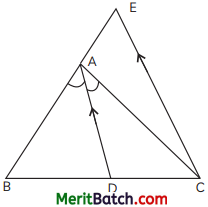To Prove: $$\frac{BD}{CD}=\frac{A B}{AC}$$
Construction: Draw CE || AD to meet BA produced at E.
Proof: Now, CE || DA to meet BA produced at E.
∠DAC = ∠ACE (alternate angles) …(i)
∠BAD = ∠AEC (corresponding angles) ………(ii)
Now, AD is the bisector of ∠A
From (i), (ii) & (iii), we get
∠ACE = ∠AEC
∴ AE = AC (Sides opposite to equal angles)

∴ In DBCE,
CE || DA
$$\frac{BD}{CD}=\frac{BA}{AE}$$ (by thales therem)
$$\frac{BD}{CD}=\frac{A B}{AC}$$ (∵ AE = AC)
Hence, Proved

Question 24.
Prove that $$\frac{1}{1+\sin \theta}+\frac{1}{1-\sin \theta}=2 \sec ^2 \theta$$Question 25.
If a and b are the zeroes of the quadratic polynomial 2x2 – 13x + 6, then find the values of:
(A) a – b
(B) a3 + b3.
OR
The angles of a triangular shaped shelf are in AP. The greatest angle is twice the smallest angle. Find all the three angles of the triangular shaped shelf.
Given; quadratic polynomial is 2x2 – 13x + 6.
Its zeroes are a and b.
a + b = -(-$$\frac{13}{2}$$) = $$\frac{13}{2}$$
ab = $$\frac{6}{2}$$ = 3
(A) (a – b)2 = (a + b)2 – 4ab
= ($$\frac{13}{2}$$)2 – 4 × 3
= $$\frac{169}{4}$$ – 12
= $$\frac{169-48}{4}$$ = $$\frac{121}{4}$$
∴ a – b = $$\frac{11}{2}$$

(B) a3 + b3 = (a + b)3 – 3ab (a + b)
= ($$\frac{13}{2}$$)2 – 3 × 3($$\frac{13}{2}$$)
= $$\frac{2197}{8}$$ – $$\frac{117}{2}$$
= $$\frac{2197-468}{8}$$ = $$\frac{1729}{8}$$

OR

Let, the three angles of an AP be (a – d), a and (a + d)
∴ a – d + a + a + d = 180
3a = 180°
⇒ a = 60°
Then, angles are (60 – d), 60, (60 + d)
According to Question,
60 + d = 2(60 – d)
⇒ 60 + d = 120 – 2d
⇒ 3d = 60
d = 20
Hence, the angles are 40°, 60° and 80°.Question 26.
Solve the equation for x : 1 + 4 + 7 + 10 + ……. x = 287 (3)
Given: 1 + 4 + 7 + 10 + …… + x = 287
Here, a = 1, common diffrence, d = 4 – 1 = 3
and Sn = 287
Now,
Sn = $$\frac{n}{2}$$[2a + (n – 1)d]
⇒ 287 = $$\frac{n}{2}$$[2 × 1 + (n – 1)3]
⇒ 574 = n(3n – 1)
⇒ 3n2 – n – 574 = 0
On solving the quadratic equation using
$$n=\frac{-b \pm \sqrt{b^2-4 a c}}{2 a}$$
we get, n = 14 and – $$\frac{41}{3}$$ (does not exist)
So, n = 14
Now, Sn = $$\frac{n}{2}$$[a+l]
287 = $$\frac{14}{2}$$(1 + x)
⇒ $$\frac{287}{7}$$ = (1 + x)
⇒ 1 + x = 41
x = 40
Hence, the value of x is 40.

Question 27.
A ceiling fan has three blades. Find the length of arc described between two consecutive blades, where the length of each blade is 0.98 m.
If the wings are equally placed, then the angle
subtended by two consecutive wings is $$\frac{360°}{3}$$ = 120°
Length of arc = $$\frac{θ}{360°}$$ × 2πr
= $$\frac{120°}{360°}$$ × 2π × 0.98
= 2 × $$\frac{22}{7×3}$$ × 0.98
= $$\frac{44}{3}$$ × 0.14
= 2.05 m (approx)

Question 28.
A vertical tower subtends a right angle at the top of a vertical flag on the ground, the height of the flag being 10 m. If the distance between the tower and the flag be 10 m, find the height of the tower.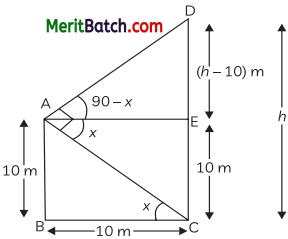Let AB be a flgstaf and CD be the vertical tower.
Let, the height of the tower, CD = h
∴ AB = EC = 10 m
Distance between flgstaf and tower = 10m
In ∆ABC,
tan x = $$\frac{AB}{BC}=\frac{10}{10}=1$$ ……(i)
tan x = tan 45°
x = 45°

tan (90 – x) = $$\frac{DE}{AE}$$
tan (90 – 45°) = $$\frac{h-10}{10}$$ (∵ AE = BC)
cot 45° = $$\frac{h-10}{10}$$
1 = $$\frac{h-10}{10}$$
h – 10 = 10
h = 20mQuestion 29.
Classroom P has 12 benches, Q has 18 benches and R has 24 benches. In each classroom, the benches are arranged in rows with the same number of benches in each row. What is greatest possible number of benches in each row in the three classrooms.
OR
A ship is at a distance of 2C0 m from a tall tower. What is the angle of depression (to the nearest degree) of the ship found by a man after climbing 112 m up the tower.
For fiding the greatest possible number of benches in each row in the 3 rooms, we have to fid the HCF of 12, 18 and 24.
∴ 12 = 22 × 3
18 = 2 × 32
24 = 22 × 3
Then HCF = 2 × 3 = 6
Hence, the greatest possible no. of benches in each row is 6.

OR

Let AB be a tower and ship is at a point D, such
that BD = 200 m.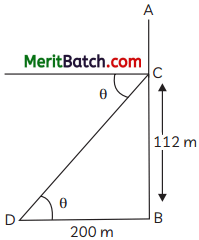Now, man is at point C on the tower, BC = 112 m
Let, θ be the angle of depression.
∴ In ∆BCD,
tan θ = $$\frac{BC}{BD}$$
= $$\frac{112}{200}$$ = $$\frac{56}{100}$$
= $$\frac{14}{25}$$
∴ θ = tan-1 ($$\frac{14}{25}$$)
Hence, the required angle of depression is tan-1 ($$\frac{14}{25}$$)

Question 30.
Evaluate distance between the points P(a sin a, -b cos a) and Q(-a cos a, b sin a)
The given points are
(a sin a, – b cos a) and 2(-a cos a, b sin a)
Here, x1 = a sin a, y1 = – b cos a
x2 = – a cos a, y2 = b sin a
By distance formula,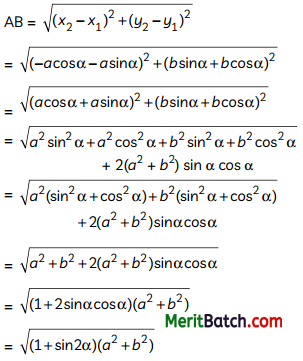Question 31.
Draw the graphs of the equations: x + y – 5 = 0; x – y – 5 = 0 and write the solution of the pair. Also, shade the triangular region formed by these lines and the y – axis.
OR
Each square on a chess board measures 6.25 square centimeters and has 64 equal squares. A border round the board is 2 cm wide. Determine length of the side of the chess board.
Given, pair of equations are:
x + y – 5 = 0 …(i)
x – y – 5 = 0 …(ii)
For equation (i)
y = 5 – x

 x 0 2 4 y 5 3 1

For equation (ii)
y = x – 5

 x 4 3 0 y -1 -2 -5Coordinates of ∆ABC are A(0, 5) B(5, 0), C(-5, 0)
Here, ar (∆ABC) = $$\frac{1}{2}$$ × OB × AC
= $$\frac{1}{2}$$ × 5 × 10
= 25 unit2
OR
Let the length of the side of the chess board be ‘x’ cm.
Then, area of 64 squares = (x – 4)2
(x – 4)2 = 64 × 6.25
⇒ x2 – 8x + 16 = 400
⇒ x2 – 8x – 384 = 0
⇒ x2 – 24x + 16x – 384 = 0
⇒ (x – 24) (x + 16) = 0
⇒ x = 24 (x = -16)
Hence, the length of the side of the chess board is 24 cm.

Section – D
(Section D consists of 4 questions of 5 mark each)

Question 32.
Every year, a factory’s output grew by the same amount. Find the percentage if it is known that the output is doubled in the last 2 years. (5)
Let P be the initial production (2 years ago) and
let the increase in product on every year be x%
Then, production at the end of fist year
= P + $$\frac{px}{100}$$
= P (1+$$\frac{x}{100}$$)
Production at the end of the second year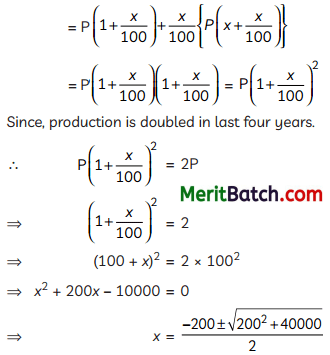⇒ x = -100 + 100$$\sqrt{2}$$
⇒ = 100(−1 ± $$\sqrt{2}$$)
= 100(−1 + $$\sqrt{2}$$)
(as negative value is not possible)
= 41.42%Question 33.
If Pn = sinn θ + cosn θ (n > 1), then prove that $$\frac{P_3-P_5}{P_1}=\frac{P_5-P_7}{P_3}$$. (5)
OR
A parallelogram, PQRS is inside a AABC in which AB || PS. Prove that OC || SR.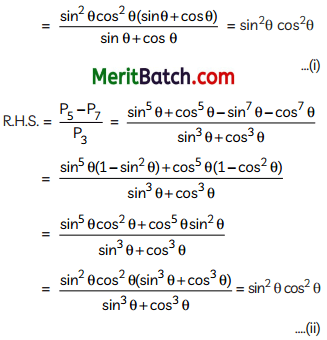From (i) and (ii),
L.H.S.= R.H.S.
Hence, Proved.

OR

Given: PQRS is a || gm, in which AB || PS
To Prove: OC || SR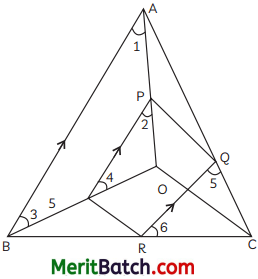Proof: In ∆OAB & ∆OPS
PS || AB (given) …(i)
∴ ∠1 = ∠2
(Corresponding Pair of angles)
∠3 = ∠4
(Corresponding Pair of angles)
∴ ∆OPS ~ ∆OAB [by AA-similarity criterion]
$$\frac{\mathrm{OP}}{\mathrm{OA}}=\frac{\mathrm{OS}}{\mathrm{OB}}=\frac{\mathrm{PS}}{\mathrm{AB}}$$ …(ii)
PQRS is a || gm, so PS || QR …(iii)
⇒ QR || AB (from (i) & (iii))
In ∆CQR & ∆CAB
∠5 = ∠CAB (Coresponding angle)
∠6 = ∠CBA (Coresponding angle)
∴ ∆CQR ~ ∆CAB (by AA similarity)
$$\frac{C Q}{C R}=\frac{C R}{C B}=\frac{Q R}{A B}$$
(Corresponding sides of similar triangle)
∴ PS = QR
∴ $$\frac{\mathrm{PS}}{\mathrm{AB}}=\frac{\mathrm{CR}}{\mathrm{CB}}=\frac{\mathrm{CQ}}{\mathrm{CA}}$$
⇒ $$\frac{\mathrm{CR}}{\mathrm{CB}}=\frac{\mathrm{OS}}{\mathrm{OB}}$$ [from (ii) & (v)]
These are the rates of two sides of ∆BOC and are equal.
So, by converse of BPT, SR || OC.
Hence, Proved.

Question 34.
A building’s interior is shaped like a right circular cylinder with a diameter of 4.2 metres and a height of 4 metres, topped by a cone. The cone has a vertical height of 2.1 m. Find the building’s volume and outer surface area. (5)
Given:
Diameter of cylinder = 4.2 cmHeight of cylinder, h = 4 m
Radius of cone (r) = 2.1 m
Height of cone, h = 2.1 m
Volume of building = volume of cone + volume cylinder
= $$\frac{1}{3}$$πr2h’ + πr2h
= πr2($$\frac{h’}{3}$$+3)
= $$\frac{22}{7}$$ × 2.1 × 2.1 + ($$\frac{2.1}{3}$$+4)
= 6.6 × 2.1 (0.7 + 4)
= 6.6 × 2.1 × 4.7
= 65.142 cm3

Outer surface area of building
= πrl + 2πrh
= πr $$\sqrt{(2.1)^2+(2.1)^2}$$ + 2π × rh
= $$\frac{22}{7}$$ × 2.1 +[3 + 2 × 4]
= 22 × 0.3 (11)
= 72.60 m2
Hence, surface area is 72.6 m2 and volume is 65.142 m3.Question 35.
In the given figure, PA, QB, RC and SD are all perpendiculars to a line l. AB = 6 cm, BC = 9 cm, CD = 12 cm and SP = 36 cm. Find the lengths of PQ, QR and RS.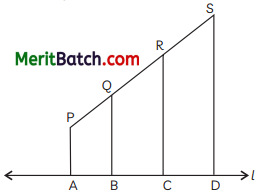OR
Find the value of k, if (a, b) ¡s the mid point of the Line segment joining the points A(1O, -6) and B(k, 4) and a – 2b = 18. Also, find the distance AB. (5)
Given: AB = 6 cm,
BC = 9 cm, CD = 12 cm and SP = 36 cm
From PA, QB, RCand SD are ⊥r the same line l, So they are parallel to each other.
By theorem of intercepts, we have
$$\frac{P Q}{A B}=\frac{Q R}{B C}=\frac{R S}{A D}=\frac{A C}{A D}$$
⇒ $$\frac{P Q}{6}=\frac{Q R}{9}=\frac{R S}{12}=\frac{36}{27}$$
⇒ PQ = $$\frac{6×36}{27}$$ = 8 cm
QR = $$\frac{9×36}{27}$$ = 12 cm
RS = $$\frac{36×12}{27}$$ = 16 cm

OR

(a, b) is the mid-point of line segment joining the points A(10, -6) and B(k, 4)
So, a = $$\frac{10k+2}{2}$$ and b = $$\frac{−6+4}{2}$$ = 1
It is given that,
a – 2b = 18
Put value of a and b
$$\frac{10k+2}{2}$$ – 2(-1) = 18
$$\frac{10k+2}{2}$$ = 16
⇒ k = 22
∴ Distance between the points A(10, -6) and B(22, 4) is:
AB = $$\sqrt{(22-10)^2 + (4+6)^2 }$$
= $$\sqrt{244}$$
= 2$$\sqrt{61}$$ units

Section – E
(Case Study Based Questions)
(Section E consists of 3 questions. All are compulsory.)

Question 36.
Sushmita along with her brother went to Diwali Mela. There she saw a game having prizes -wall clocks, power banks, puppets, water bottles and purses. The game consists of a box having number cards bearing numbers 1 to 200, one on each card. A person has to draw a card at random from the box.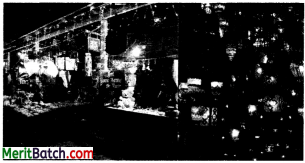Winning of prizes has the following conditions:

• Wall clock : If the number on drawn card is a perfect square.
• Power bank: If the number on drawn card is an even number which is also a multiple of 3.
• Water bottle : If the number on drawn card is a multiple of 10
• Puppet : If the number on drawn card is a perfect cube.
• Purse : If the number on drawn card is a prime number greater than 100 but Less than 200.

Based on the above, answer the following questions: (Take π=3.14)
(A) What is the probability of winning a purse and winning a wall clock ?
OR
What is the probability of winning a power bank ?
(B) What is the probability of winning a puppet ?
(C) What is the probability of winning a water bottle ?
(A) Total number of cards = 200
Favourable outcome for getting a purse
= 101, 103, 107, 109, 113, 127, 131, 137,
139, 140, 151, 157, 163, 167, 173, 179,
181, 191, 193, 197, 199 i.e. 21.

P(winning a purse) = $$\frac{21}{200}$$
Favourable outcomes of winning a wall clock
= 4, 9, 16, 25, 36, 49, 64, 81, 100, 121, 144, 169, 196, i.e. 13.
P(winning a wall clock) = $$\frac{13}{200}$$

OR

Favourable outcomes for winning a power bank are:
6, 12, 18, 24, 30, 36, 42, 48, 54,
60, 66, 72, 78, 84, 90, 96 102, 108, 114,
120 126, 132, 138, 144, 150, 156, 162,
168, 174, 180, 186, 192, 198 i.e. 33.
P(winning a power bank) = $$\frac{33}{200}$$

(B) Favourable outcomes for winning a puppet are:
1, 8, 27, 64, 125, i.e. 5
P(winning a puppeet = $$\frac{5}{200}$$ = $$\frac{1}{40}$$

(C) Favourable outcomes of winning a water bottle are:
10, 20, 30, 40, 50, 60, 70, 80, 90,
100, 110, 120, 130, 140, 150, 160, 170,
180, 190, 200 i.e. 20

P(winning a water bottle) = $$\frac{20}{200}$$
= $$\frac{1}{10}$$

(E) (d) $$\frac{33}{200}$$Question 37.
A welfare society of birds constructed a circular tank in quadilateral shaped field to serve as a bird bath as shown in the figure below:
Here, ABCD is a quadrilateral where in sides AB, BC, CD and DA act as tangents to circle at E, F, G and H. Here, AB = 5 m, DC = 6 m and BC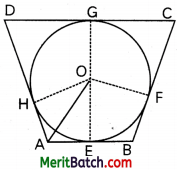Based on the above, answer the following questions:
(A) What is the length of the side AD?
OR
If ∠GOF = (3x – 8)° and ∠GCF = (2x + 3)°, then what is the value of x ?
(B) if O is the centre of tank and AH and AE are inclined to each other at an angle of 100°, then what is the measure of angle HOE?
(C) What kind of triangle OHA is ?
(A) Since, length of tangents from an external
point are equal.
∴ AE = AH …(i)
EB = BF …(ii)
GC = CF …(iii)
DG = DH …(iv)
AE + EB + GC + DG = AH + BK + CF + DH
(on adding (i), (ii), (iii) and (iv))
⇒ AB + CD = BC + AD
⇒ 5 + 6 = 7 + AD

OR

Given,
∠GOF = (3x – 8), ∠GCF = (2x + 3)
∠OGC = ∠OFC = 90°
(Since, tangent is ⊥ to radius)
∴ ∠GOF + ∠GCF = 180°
3x – 8 + 2x + 3 = 180°
⇒ 5x – 5 = 180°
⇒ 5x = 185°
x = 37°

(B) Here,
∠HAE = 100°
∠OHA = ∠OEA = 90°
(∵ Tangent is ⊥r to the radii)
∠HOE +∠OHA +∠OEA +∠HAE = 360°
90° + 90° + 100° + ∠HOE = 360°
∠HOE = 360° – 280°
= 80°

(C) In ∆OHA,
∠OHA = 90°, as tangent is ⊥ to the radius
∴ DOHA is a right – angled triangle.Question 38.
Direct income in India was drastically impacted due to the COVID- 19 lockdown. Most of the companies decided to bring down the salaries of the employees upto 50%.The following table shows the salaries (in %) received by 50 employees during lockdown:

 Salary received (in %) 50-60 60-70 70-80 80-90 Number of employees 18 12 16 4

Based on the above information, answer the following questions:
(A) Rnd the cumulative frequencies of given data.
OR
What is the mean salary received (in %) of 50 employees?
(B) What is the number of employees whose salary was reduced by more than 30%?
(C) What is the number of employees whose salary was reduced by at most 40%?
(A)

 Salary recieved (in%) No. of employees c.f 50 – 60 18 18 60 – 70 12 30 70 – 80 16 46 80 – 90 4 50

OR

 xi fi fixi 55 18 990 65 12 780 75 16 1200 85 4 340 Total 50 3310

∴ Mean = $$\frac{Σf_i x_i}{Σf_i}$$
= $$\frac{3310}{50}$$
= 66.2
(B) Reduction of more than 30% means recieving 70% of salary.
(C) Employees with reduction in salary of at most 40% = 12 + 16 + 4
= 32